# Acceleration wave

Jump to: navigation, search

In the mechanics of continuous media, the behaviour of a material body is described by a number of field variables which are required to satisfy a set of governing partial differential equations arising from balance laws, and from kinematic and constitutive considerations. The variables are generally assumed to have the requisite degree of smoothness consistent with the governing equations, except possibly on surfaces in the body across which some of the variables may suffer jump discontinuities (cf. also Smooth function).

Suppose that a material body occupies a regioninat time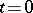, and at some later timeoccupies, in its deformed state, a region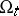. The motion of the body is described by the function,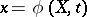, in whichdenotes the position at timeof a material particle, andits position at time. The functionis assumed to be invertible, and bothand its inverse are assumed to be continuously differentiable with respect to the spatial and temporal variables on which they depend, except possibly on specified surfaces in the body. A propagating smooth surface divides the body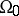orinto two regions, forming a common boundary between them. The unit normalto the surface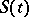is considered to be in the direction in whichpropagates. The region ahead of the surface is denoted byand the region behind the surface is denoted by. Letbe an arbitrary scalar-, vector- or tensor-valued function which is continuous in both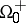and. This function has definite limits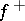and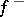at a point on, as the point is approached fromand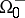. The jump ofatis defined by(a1)

The surfaceis called a singular surface with respect toat timeif. A singular surface that has a non-zero normal velocity called a wave. An acceleration wave is a propagating singular surface across which the motion, velocityand (hence) the deformation gradient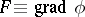, are continuous; however, quantities involving second-order derivatives of the motion, such as the acceleration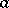and the time rate of deformation gradient, are discontinuous. Various kinematical and geometrical conditions of compatibility involving the variablesand, the normalto the surface, and the speedof the surface, may be derived with the aid of Hadamard's lemma (see, for example, [a2], [a4]). These considerations lead to the propagation condition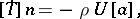(a2)

which is a statement of balance of linear momentum across the surface;is the Cauchy stress,is the mass density, andis the intrinsic speed of the surface. To make further progress it is necessary to introduce information about the constitution of the material; in the case of an elastic material, for example, (a2) becomes the eigenvalue problem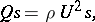(a3)

in whichis referred to as the amplitude vector of the acceleration jump, andis the acoustic tensor. This leads to the Fresnel–Hadamard theorem: The amplitudeof an acceleration wave travelling in the directionmust be an eigenvector of the acoustic tensor; the corresponding eigenvalue is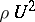. It follows that, for real wave speeds to exist,must possess at least one real and positive eigenvalue. The acoustic tensor is symmetric, and consequently its eigenvalues are real, if and only if the material is hyperelastic. In addition,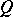possesses three positive eigenvalues if and only if it is positive definite; in the context of elasticity, positive definiteness ofimplies that the material is strongly elliptic. Further information on acceleration waves may be found in the references cited.